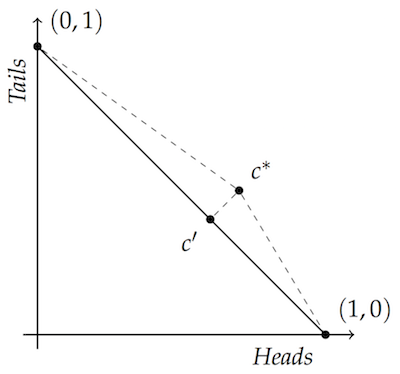# Accuracy for Dummies, Part 1: Euclid Improper

If you’ve bumped into the accuracy framework before, you’ve probably seen a diagram like this one:The vertices $(1,0)$ and $(0,1)$ represent two possibilities, whether a coin lands heads or tails in this example.

# Where This Leaves Us

Wait a minute: if Euclidean distance is a bad way to measure inaccuracy, then what’s the use of the diagram we started with?? And what’s the right way to measure inaccuracy?

We’ll tackle these questions in the next post. But here’s the short answer.

One common way of measuring inaccuracy is a variation on Euclidean distance called Brier distance. Brier distance is just enough like Euclidean distance to vindicate the reasoning we did with our opening diagram. But it’s different enough from Euclidean distance to avoid the instability problem we ended up with.

So what is Brier distance? It’s just the square of Euclidean distance. Just take the square root symbol off Euclid’s formula and you’ve got the formula for Brier distance. Next time we’ll see how that one change makes all the right differences.